# Electronic Devices - Op-Amp Applications

### Exercise :: Op-Amp Applications - General Questions

6.

Calculate the output voltage if V1 = V2 = 0.15 V.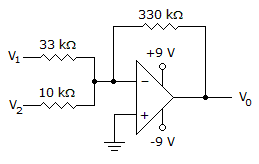A. 0 V B. 4.65 V C. 6.45 V D. –6.45 V

Explanation:

No answer description available for this question. Let us discuss.

7.

Determine the output voltage for this circuit with a sinusoidal input of 2.5 mV.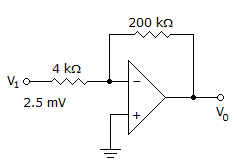A. –0.25 V B. –0.125 V C. 0.25 V D. 0.125 V

Explanation:

No answer description available for this question. Let us discuss.

8.

Determine the output voltage when V1 = V2 = 1 V.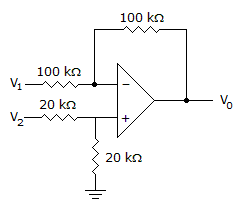A. 0 V B. –2 V C. 1 V D. 2 V

Explanation:

No answer description available for this question. Let us discuss.

9.

How many op-amps are required to implement this equation?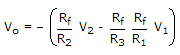A. 2 B. 3 C. 4 D. 1

Explanation:

No answer description available for this question. Let us discuss.

10.

Calculate the input voltage if the final output is 10.08 V.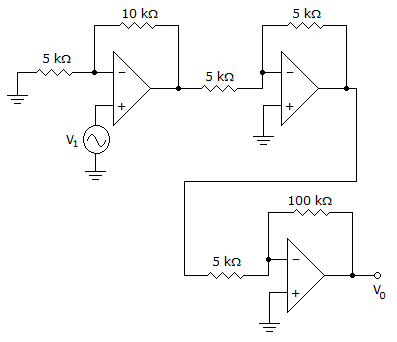A. –1.05 V B. 0.525 V C. 0.168 V D. 4.2 V